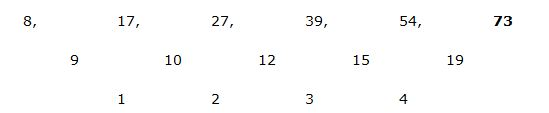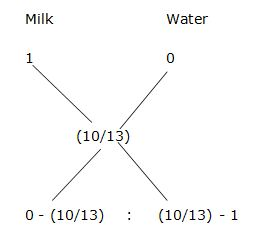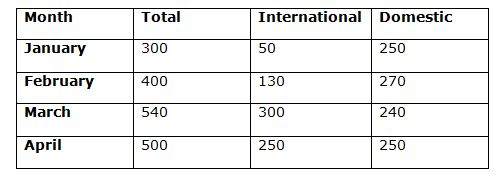# “20-20” Quantitative Aptitude | Crack IBPS RRB Assistant Prelims 2018 Day-167

Dear Readers, Find the aptitude test questions to crack latest bank exams. We regularly provide 20 aptitude test questions daily for students. Aspirants practice these questions on a regular basis to improve your score in aptitude section. Aspirants preparing for the exams can make use of this “20-20” Quantitative Aptitude Questions. Here we have started New Series of Practice Materials specially for IBPS RRB Assistant Prelims 2018. Aspirants those who are preparing for the exams can use this “20-20” Quantitative Aptitude Questions.

“20-20” Aptitude Questions | Crack IBPS RRB Assistant Prelims 2018 Day-167

Click “Start Quiz” to attend these Questions and view Solutions

Directions (1 – 5): What value should come in the place of question mark (?) in the following questions?

1) 48 % of 3850 + 16 % of 750 + 21 % of? = 2115

1. 550
2. 800
3. 700
4. 1050
5. None of these

2) ? % of 800 = 2560 – (522 – 272) × 36 ÷ 395

1. 297.5
2. 356.75
3. 154.25
4. 412.5
5. None of these

3) 54 × 3 × (34 – 11) + 216 ÷ 4 ÷ 3 – 562 =?

1. 524
2. 356
3. 790
4. 608
5. None of these

4) 50653 – 17576 % of 350 + 1404928 % of 800 = ?

1. 576
2. 842
3. 620
4. 784
5. None of these

5) (28928 ÷ 113) + (5249 ÷ 29) + (2584 ÷ 17) + (3157 ÷ 7) = ?

1. 1040
2. 1250
3. 1370
4. 1180
5. None of these

Directions (6 – 10): What value should come in the place of question mark (?) in the following number series?

6)  8, 17, 27, 39, 54, ?

1. 82
2. 73
3. 91
4. 105
5. 66

7) 23, 12, 13, 21, ? , 112.5

1. 56
2. 38
3. 29
4. 44
5. 63

8) 85, 97, 117, 147, 189, 245, ?

1. 317
2. 356
3. 389
4. 412
5. 438

9) 13, 26, 104, 624, ? , 49920

1. 3785
2. 3924
3. 4992
4. 4578
5. 4620

10) 7, 15, 63, 383, 3071, ?

1. 12565
2. 30719
3. 28567
4. 22478
5. 18355

11) A boat covers 32 km in 4 hours along the stream and 20 km in 5 hours against the stream. Find the speed of stream

1. 5 km/hr
2. 8 km/hr
3. 2 km/hr
4. 6 km/hr
5. None of these

12)  In what ratio water be mixed with milk to earn a profit of 30 % by selling the mixture at cost price?

1. 5 : 6
2. 9 : 7
3. 3 : 5
4. 2 : 7
5. 10 : 3

13) Priyanka bought a microwave oven and paid 10% less than its original price. She sold it at 30% profit on the price she paid. What percentage of profit did she earn?

1. 29
2. 18
3. 20
4. 27
5. 16

14) A, B and C started a business with their investments in the ratio 1: 2:4. After 6 month A invested the half amount more as before and B invested the same amount as before while C withdrew ¼ part of his investment. Find the ratio of their profits at the end of the year.

1. 5: 12: 13
2. 5: 11: 14
3. 5: 12: 14
4. 5: 12: 10
5. None of these

15) 6 women and 6 men can do a work in 8 days. 5 women and 9 men can do a same work in 6 days. How long will 6 women and 8 men can take to do the work?

1. 6 2/5 days
2. 7 ½ days
3. 5 ¾ days
4. 8 ¼ days
5. None of these

Directions (16-20): Read the following passage carefully and answer the given questions

In a survey conducted by airways, no of international and domestic flight runs in four different months. Total no of flights in January is same as the no of international flights in March. A total flight in March is twice the no of domestic flights in February and the no of international flights in February is 130 which is 30 more than twice the no of international flights in January. In April, no of international and domestic flights divided equally which is equal to the no of domestic flights in January which is equal to 250. The ratio of no of domestic flights in February to March is 9:8 and the no of domestic flights in February is 20 more than the no of domestic flights in April.

16) What is the difference between the total flights in January and total flights in February?

1. 120
2. 150
3. 110
4. 100
5. 80

17) Find the total number of international flights for all the given months together.

1. 750
2. 720
3. 730
4. 700
5. 840

18) Find the ratio of the no of international flights in January and February together to the no of domestic flights in February?

1. 1:3
2. 2:3
3. 4:3
4. 2:5
5. 1:2

19) What is the ratio of the difference between the no of international flights in January and February to the difference between the no of international flights in March and April?

1. 4:3
2. 6:7
3. 9:8
4. 7:5
5. 8:5

20) What is the difference between the average no of international flights and average no of domestic flights for all the given months together?

1. 80
2. 90
3. 70
4. 65
5. 85

Direction (1-5)

(48/100)*3850 + (16/100)*750 + (21/100)*x = 2115

1848 + 120 + (21/100)*x = 2115

(21/100)*x = 2115 – 1848 -120

(21/100)*x = 147

X = 147*100/21 = 700

(x/100)*800 = 2560 – [(52 + 27) (52 – 27) × 36 ÷ 395]

8x = 2560 – [79*25*36/395]

8x = 2560 – 180

8x = 2380

X = (2380/8) = 297.5

(54*3*23) + 216 ÷ 4 ÷ 3 – 562 = x

3726 + 18 – 3136 = x

X = 608

37 – 26 % of 350 + 112 % of 800 = x

37 – (26/100)*350 + (112/100)*800 = x

37 – 91 + 896 = x

X = 842

(28928/113) + (5249/29) + (2584/17) + (3157/7) = x

X = 256 + 181 + 152 + 451

X = 1040

Direction (6-10):The pattern is, *0.5 + 0.5, *1 + 1, *1.5 + 1.5, *2 + 2, *2.5 + 2.5,..

The pattern is, +(3*4), +(4*5), +(5*6), +(6*7), +(7*8), +(8*9),…

The pattern is, *2, *4, *6, *8, *10

The pattern is, *2 + 1, *4 + 3, * 6 + 5, *8 + 7, *10 + 9,..

Speed of downstream = 32/4 = 8 km/hr

Speed of upstream = 20/5 = 4 km/hr

Speed of stream = (1/2) (Speed of downstream – Speed of upstream)

= > (1/2) (8 – 4) = 4/2 = 2 km/hr

Let the cost price be Rs. 1

Selling price of mixture = Rs. 1

Cost price of mixture = 1*100/130 = 10/13= > – (10/13) : – (3/13)

= > 10 : 3

Let the original price be Rs. 100

Money paid by Priyanka = 100*90/100= Rs. 90

Priyanka sold at = 90*130/100= Rs. 117

Profit earned= 117 – 90 = Rs. 27

Profit percentage = 27*100/100 = 27 %

The share of A, B and C = [1*6 + (3/2)*6]: [2*6 + 4*6]: [4*6 + 4 *(3/4)*6]

= > 15: 36: 42

= > 5: 12: 14

Total work = (men (or) women)*days

Work equal, so,

(6 w + 6 m)*8 = (5 w + 9 m)*6

48 w + 48 m = 30 w + 54 m

18 w= 6 m

9 w = 3 m

3 w = 1 m

6 w + 6 m = 2 m+ 6 m = 8 m

6 w + 8 m = 2 m + 8 m = 10 m

Men            days

8                 8

10               ?

8*8 = 10x

X= 8*8/10 = 32/5 = 6 2/5 days

Directions (16-20):Required difference = 300 – 400 = 100

Required total = 50+130+300+250 = 730

Required ratio = (50+130): 270 = 180:270 =2:3

Required ratio = (130-50) : (300-250) = 80 : 50 = 8:5